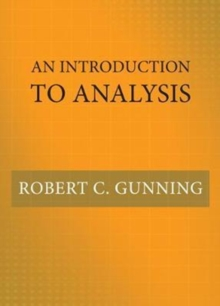Supporting your high street Find out how »
• My AccountAn Introduction to Analysis Hardback

Description

An essential undergraduate textbook on algebra, topology, and calculusAn Introduction to Analysis is an essential primer on basic results in algebra, topology, and calculus for undergraduate students considering advanced degrees in mathematics.

Ideal for use in a one-year course, this unique textbook also introduces students to rigorous proofs and formal mathematical writing--skills they need to excel. With a range of problems throughout, An Introduction to Analysis treats n-dimensional calculus from the beginning-differentiation, the Riemann integral, series, and differential forms and Stokes's theorem-enabling students who are serious about mathematics to progress quickly to more challenging topics.

The book discusses basic material on point set topology, such as normed and metric spaces, topological spaces, compact sets, and the Baire category theorem.

It covers linear algebra as well, including vector spaces, linear mappings, Jordan normal form, bilinear mappings, and normal mappings. Proven in the classroom, An Introduction to Analysis is the first textbook to bring these topics together in one easy-to-use and comprehensive volume. Provides a rigorous introduction to calculus in one and several variablesIntroduces students to basic topologyCovers topics in linear algebra, including matrices, determinants, Jordan normal form, and bilinear and normal mappingsDiscusses differential forms and Stokes's theorem in n dimensionsAlso covers the Riemann integral, integrability, improper integrals, and series expansions

Information

• Format: Hardback
• Pages: 384 pages, 25 b/w illus.
• Publisher: Princeton University Press
• Publication Date:
• Category: Calculus & mathematical analysis
• ISBN: 9780691178790

Other Formats

£62.00

£46.45

on all orders

Pick up orders

from local bookshops

£45.00

£44.15

£62.00

£61.99

£24.00

£18.15

£24.00

£18.15